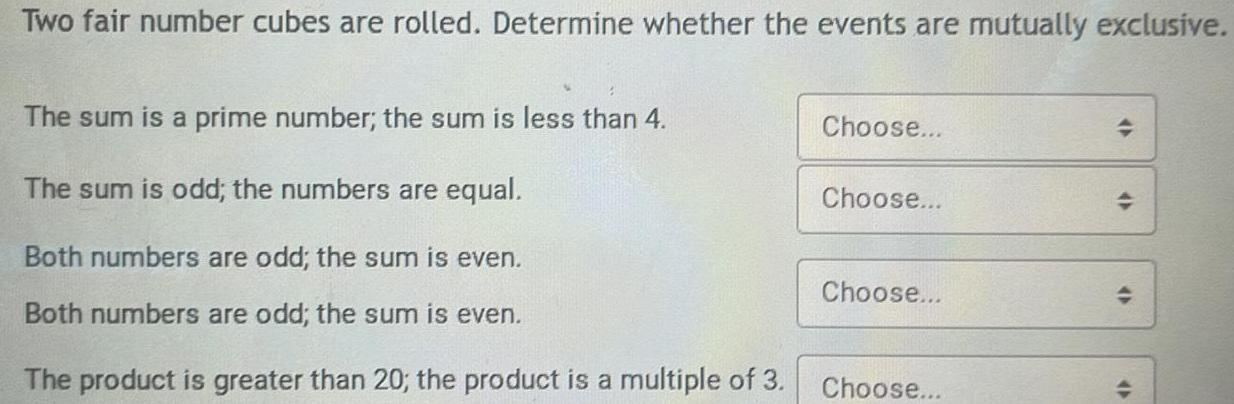Question:

# Two fair number cubes are rolled. Determine whether the

Last updated: 7/7/2022Two fair number cubes are rolled. Determine whether the events are mutually exclusive. The sum is a prime number; the sum is less than 4. The sum is odd; the numbers are equal. Both numbers are odd; the sum is even. Both numbers are odd; the sum is even. The product is greater than 20; the product is a multiple of 3.